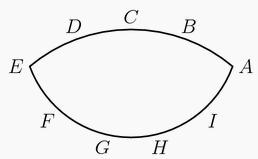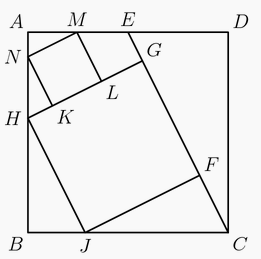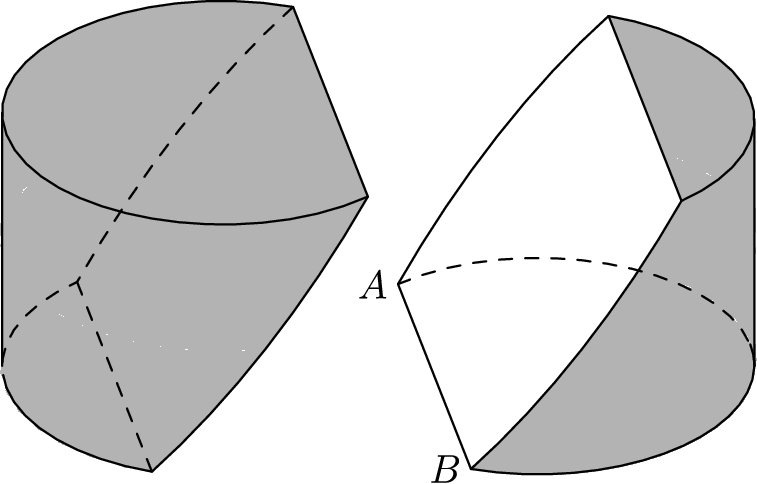US

back to index

Solve in integers the equation $$x^2+xy+y^2 = \left(\frac{x+y}{3}+1\right)^3.$$

Quadrilateral $APBQ$ is inscribed in circle $\omega$ with $angle P = \angle Q = 90^{\circ}$ and $AP = AQ < BP$. Let $X$ be a variable point on segment $\overline{PQ}$. Line $AX$ meets $\omega$ again at $S$ (other than $A$). Point $T$ lies on arc $AQB$ of $\omega$ such that $\overline{XT}$ is perpendicular to $\overline{AX}$. Let $M$ denote the midpoint of chord $\overline{ST}$. As $X$ varies on segment $\overline{PQ}$, show that $M$ moves along a circle.

Consider $0<\lambda<1$, and let $A$ be a multiset of positive integers. Let $A_n={a\in A: a\leq n}$. Assume that for every $n\in\mathbb{N}$, the set $A_n$ contains at most $n\lambda$ numbers. Show that there are infinitely many $n\in \mathbb{N}$ for which the sum of the elements in $A_n$ is at most $\frac{n(n+1)}{2}\lambda$. (A multiset is a set-like collection of elements in which order is ignored, but repetition of elements is allowed and multiplicity of elements is significant. For example, multisets ${1, 2, 3}$ and ${2, 1, 3}$ are equivalent, but ${1, 1, 2, 3}$ and ${1, 2, 3}$ differ.)

The expressions $A$ = $1\times 2 + 3\times 4 + 5\times 6 + \cdots + 37 \times 38 + 39$ and $B$ = $1 + 2 \times 3 + 4\times 5 + \cdots + 36\times 37 + 38\times 39$ are obtained by writing multiplication and addition operators in an alternating pattern between successive integers. Find the positive difference between integers $A$ and $B$.

The nine delegates to the Economic Cooperation Conference include $2$ officials from Mexico, $3$ officials from Canada, and $4$ officials from the United States. During the opening session, three of the delegates fall asleep. Assuming that the three sleepers were determined randomly, find the probability that exactly two of the sleepers are from the same country.

There is a prime number $p$ such that $16p+1$ is the cube of a positive integer. Find $p$.

Point $B$ lies on line segment $\overline{AC}$ with $AB=16$ and $BC=4$. Points $D$ and $E$ lie on the same side of line $AC$ forming equilateral triangles $\triangle ABD$ and $\triangle BCE$. Let $M$ be the midpoint of $\overline{AE}$, and $N$ be the midpoint of $\overline{CD}$. The area of $\triangle BMN$ is $x$. Find $x^2$.

In a drawer Sandy has $5$ pairs of socks, each pair a different color. On Monday Sandy selects two individual socks at random from the $10$ socks in the drawer. On Tuesday Sandy selects $2$ of the remaining $8$ socks at random and on Wednesday two of the remaining $6$ socks at random. Find the probability that Wednesday is the first day Sandy selects matching socks.

Point $A,B,C,D,$ and $E$ are equally spaced on a minor arc of a cirle. Points $E,F,G,H,I$ and $A$ are equally spaced on a minor arc of a second circle with center $C$ as shown in the figure below. The angle $\angle ABD$ exceeds $\angle AHG$ by $12^\circ$. Find the degree measure of $\angle BAG$.In the diagram below, $ABCD$ is a square. Point $E$ is the midpoint of $\overline{AD}$. Points $F$ and $G$ lie on $\overline{CE}$, and $H$ and $J$ lie on $\overline{AB}$ and $\overline{BC}$, respectively, so that $FGHJ$ is a square. Points $K$ and $L$ lie on $\overline{GH}$, and $M$ and $N$ lie on $\overline{AD}$ and $\overline{AB}$, respectively, so that $KLMN$ is a square. The area of $KLMN$ is $99$. Find the area of $FGHJ$.For positive integer $n$, let $s(n)$ denote the sum of the digits of $n$. Find the smallest positive integer satisfying $s(n) = s(n+864) = 20$.

Let $S$ be the set of all ordered triple of integers $(a_1,a_2,a_3)$ with $1 \le a_1,a_2,a_3 \le 10$. Each ordered triple in $S$ generates a sequence according to the rule $a_n=a_{n-1}\cdot | a_{n-2}-a_{n-3} |$ for all $n \ge 4$. Find the number of such sequences for which $a_n=0$ for some $n$.

Let $f(x)$ be a third-degree polynomial with real coefficients satisfying $$|f(1)|=|f(2)|=|f(3)|=|f(5)|=|f(6)|=|f(7)|=12.$$ Find $|f(0)|$.

Triangle $ABC$ has positive integer side lengths with $AB=AC$. Let $I$ be the intersection of the bisectors of $\angle B$ and $\angle C$. Suppose $BI=8$. Find the smallest possible perimeter of $\triangle ABC$.

Consider all $1000$-element subsets of the set $\{1, 2, 3, ... , 2015\}$. From each such subset choose the least element. Find the arithmetic mean of all of these least elements.

With all angles measured in degrees, the product $\displaystyle\prod_{k=1}^{45} csc^2(2k-1)^\circ=m^n$, where $m$ and $n$ are integers greater than 1. Find $m+n$.

For each integer $n \ge 2$, let $A(n)$ be the area of the region in the coordinate plane defined by the inequalities $1\le x \le n$ and $0\le y \le x \left\lfloor \sqrt x \right\rfloor$, where $\left\lfloor \sqrt x \right\rfloor$ is the greatest integer not exceeding $\sqrt x$. Find the number of values of $n$ with $2\le n \le 1000$ for which $A(n)$ is an integer.

A block of wood has the shape of a right circular cylinder with radius $6$ and height $8$, and its entire surface has been painted blue. Points $A$ and $B$ are chosen on the edge of one of the circular faces of the cylinder so that $\overset\frown{AB}$ on that face measures $120^\text{o}$. The block is then sliced in half along the plane that passes through point $A$, point $B$, and the center of the cylinder, revealing a flat, unpainted face on each half. The area of one of these unpainted faces is $a\cdot\pi + b\sqrt{c}$, where $a$, $b$, and $c$ are integers and $c$ is not divisible by the square of any prime. Find $a+b+c$.Let $N$ be the least positive integer that is both $22$ percent less than one integer and $16$ percent greater than another integer. Find the remainder when $N$ is divided by $1000$.

In a new school $40$ percent of the students are freshmen, $30$ percent are sophomores, $20$ percent are juniors, and $10$ percent are seniors. All freshmen are required to take Latin, and $80$ percent of the sophomores, $50$ percent of the juniors, and $20$ percent of the seniors elect to take Latin. Find the probability that a randomly chosen Latin student is a sophomore.

Let $m$ be the least positive integer divisible by $17$ whose digits sum is $17$. Find $m$.

In an isosceles trapezoid, the parallel bases have lengths $log 3$ and $log 192$, and the altitude to these bases has length $log 16$. The perimeter of the trapezoid can be written in the form $log 2^p 3^q$, where $p$ and $q$ are positive integers. Find $p + q$.

Two unit squares are selected at random without replacement from an $n \times n$ grid of unit squares. Find the least positive integer $n$ such that the probability that the two selected unit squares are horizontally or vertically adjacent is less than $\frac{1}{2015}$.

Steve says to Jon, 'I am thinking of a polynomial whose roots are all positive integers. The polynomial has the form $$P(x) = 2x^3-2ax^2+(a^2-81)x-c$$

for some positive integers $a$ and $c$. Can you tell me the values of $a$ and $c$?' After some calculations, Jon says, 'There is more than one such polynomial.' Steve says, 'You're right. Here is the value of $a$.' He writes down a positive integer and asks, 'Can you tell me the value of $c$?' Jon says, 'There are still two possible values of $c$.' Find the sum of the two possible values of $c$.

Triangle $ABC$ has side lengths $AB = 12$, $BC = 25$, and $CA = 17$. Rectangle $PQRS$ has vertex $P$ on $\overline{AB}$, vertex $Q$ on $\overline{AC}$, and vertices $R$ and $S$ on $\overline{BC}$. In terms of the side length $PQ = w$, the area of $PQRS$ can be expressed as the quadratic polynomial Area($PQRS$) = $\alpha w - \beta \cdot w^2$. Then the coefficient $\beta = \frac{m}{n}$, where $m$ and $n$ are relatively prime positive integers. Find $m+n$.